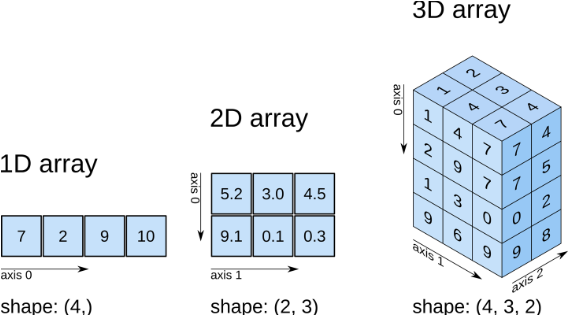## 使用MXNet的NDArray来处理数据

2018-03-06 14:29  ☆Ronny丶  阅读(10885)  评论(0编辑  收藏

NDArray.ipynb## NDArray介绍

MXNet的NDArray与Numpy中的ndarray极为相似，NDAarray为MXNet中的各种数学计算提供了核心的数据结构，NDArray表示一个多维的、固定大小的数组，并且支持异构计算。那为什么不直接使用Numpy呢？MXNet的NDArray提供额外提供了两个好处：

• 支持异构计算，数据可以在CPU,GPU，以及多GPU机器的硬件环境下高效的运算
• NDArray支持惰性求值，对于复杂的操作，可以在有多个计算单元的设备上自动的并行运算。

## NDArray的重要属性

• ndarray.shape：数组的维度。它返回了一个整数的元组，元组的长度等于数组的维数，元组的每个元素对应了数组在该维度上的长度。比如对于一个n行m列的矩阵，那么它的形状就是(n,m)。
• ndarray.dtype：数组中所有元素的类型，它返回的是一个numpy.dtype的类型，它可以是int32/float32/float64等，默认是'float32'的。
• ndarray.size：数组中元素的个数，它等于ndarray.shape的所有元素的乘积。
• ndarray.context：数组的存储设备，比如：cpu()gpu(1)
import mxnet as mx
import mxnet.ndarray as nd

a = nd.ones(shape=(2,3),dtype='int32',ctx=mx.gpu(1))
print(a.shape, a.dtype, a.size, a.context)


## NDArray的创建

1. 使用ndarray.array直接将一个list或numpy.ndarray转换为一个NDArray
2. 使用一些内置的函数zeros,ones以及一些随机数模块ndarray.random创建NDArray，并预填充了一些数据。
3. 从一个一维的NDArray进行reshape
import numpy as np

l = [[1,2],[3,4]]
print(nd.array(l)) # 从List转到NDArray
print(nd.array(np.array(l))) # 从np.array转到NDArray

# 直接利用函数创建指定大小的NDArray
print (nd.zeros((3,4), dtype='float32'))
print (nd.ones((3,4), ctx=mx.gpu()))
# 从一个正态分布的随机数引擎生成了一个指定大小的NDArray，我们还可以指定分布的参数，比如均值，标准差等
print (nd.random.normal(shape=(3,4)))
print (nd.arange(18).reshape(3,2,3))


## NDArray的查看

a = nd.random.normal(0, 2, shape=(3,3))
print(a)
print(a.asnumpy())


## 基本的数学运算

NDArray之间可以进行加减乘除等一系列的数学运算，其中大部分的运算都是逐元素进行的。

shape=(3,4)
x = nd.ones(shape)
y = nd.random_normal(0, 1, shape=shape)
x + y # 逐元素相加
x * y # 逐元素相乘
nd.exp(y) # 每个元素取指数
nd.sin(y**2).T # 对y逐元素求平方，然后求sin，最后对整个NDArray转置
nd.maximum(x,y) # x与y逐元素求最大值


nd.dot(x, y.T)


## 索引与切片

MXNet NDArray提供了各种截取的方法，其用法与Python中list的截取操作以及Numpy.ndarray中的截取操作基本一致。

x = nd.arange(0, 9).reshape((3,3))
x[1:3] # 截取x的axis=0的第1和第2行
x[1:2,1:3] # 截取x的axis=0的第1行，axis=1的第一行和第二行


## 存储变化

x = nd.ones((3,4))
y = nd.ones((3,4))
before = id(y)
y = x + y
print(before, id(y))


y += x
print(id(y))

print(id(y))

y[:] = x + y
print(id(y))


x = nd.ones((2,2))
y = x
print(id(x))
print(id(y))


y = x.copy()
print(id(y))


# 将v加到x的每一行中，并将结果存储在y中
x = nd.array([[1,2,3], [4,5,6], [7,8,9], [10, 11, 12]])
v = nd.array([1, 0, 1])
y = nd.zeros_like(x)   # Create an empty matrix with the same shape as x

for i in range(4):
y[i, :] = x[i, :] + v
print (y)


x = nd.array([[1,2,3], [4,5,6], [7,8,9], [10, 11, 12]])
v = nd.array([1, 0, 1])
vv = nd.tile(v, (4, 1))  # Stack 4 copies of v on top of each other
y = x + vv  # Add x and vv elementwise
print (y)
print(vv)


NDArray的广播机制使得我们不用像上面那样先创建vv，可以直接进行运算

x = nd.array([[1,2,3], [4,5,6], [7,8,9], [10, 11, 12]])
v = nd.array([1, 0, 1])
y = x + v
print(y)


1. 如果数组的秩不同，使用1来将秩较小的数组进行扩展，直到两个数组的尺寸的长度都一样。
2. 如果两个数组在某个维度上的长度是一样的，或者其中一个数组在该维度上长度为1，那么我们就说这两个数组在该维度上是相容的。
3. 如果两个数组在所有维度上都是相容的，他们就能使用广播。
4. 如果两个输入数组的尺寸不同，那么注意其中较大的那个尺寸。因为广播之后，两个数组的尺寸将和那个较大的尺寸一样。
5. 在任何一个维度上，如果一个数组的长度为1，另一个数组长度大于1，那么在该维度上，就好像是对第一个数组进行了复制。

## 在GPU上运算

NDArray支持数组在GPU设备上运算，这是MXNet NDArray和Numpy的ndarray最大的不同。默认情况下NDArray的所有操作都是在CPU上执行的，我们可以通过ndarray.context来查询数组所在设备。在有GPU支持的环境上，我们可以指定NDArray在gpu设备上。

gpu_device = mx.gpu(0)
def f():
a = mx.nd.ones((100,100))
b = mx.nd.ones((100,100), ctx=mx.cpu())
c = a + b.as_in_context(a.context)
print(c)

f() # 在CPU上运算

# 在GPU上运算
with mx.Context(gpu_device):
f()


## NDArray的序列化

import pickle

a = nd.ones((2,3))
data = pickle.dumps(a) # 将NDArray直接序列化为内存中的bytes

pickle.dump(a, open('tmp.pickle', 'wb')) # 将NDArray直接序列化为文件
b = pickle.load(open('tmp.pickle', 'rb')) # 从文件反序列化为NDArray


a = mx.nd.ones((2,3))
b = mx.nd.ones((5,6))
nd.save("temp.ndarray", [a, b]) # 写入与读取的路径支持Amzzon S3以及Hadoop HDFS等。


## 惰性求值与自动并行化

MXNet使用了惰性求值来追求最佳的性能。当我们在Python中运行a = b + 1时，Python线程只是将运算Push到了后端的执行引擎，然后就返回了。这样做有下面两个好处：

1. 当操作被push到后端后，Python的主线程可以继续执行下面的语句，这对于Python这样的解释性的语言在执行计算型任务时特别有帮助。
2. 后端引擎可以对执行的语句进行优化，比如进行自动并行化处理。

import time
def do(x, n):
"""push computation into the backend engine"""
return [mx.nd.dot(x,x) for i in range(n)]
def wait(x):
"""wait until all results are available"""
for y in x:

tic = time.time()
a = mx.nd.ones((1000,1000))
b = do(a, 50)
print('time for all computations are pushed into the backend engine:\n %f sec' % (time.time() - tic))
wait(b)
print('time for all computations are finished:\n %f sec' % (time.time() - tic))


a = mx.nd.ones((2,3))
b = a + 1
c = a + 2
d = b * c


n = 10
a = mx.nd.ones((1000,1000))
b = mx.nd.ones((6000,6000), gpu_device)
tic = time.time()
c = do(a, n)
wait(c)
print('Time to finish the CPU workload: %f sec' % (time.time() - tic))
d = do(b, n)
wait(d)
print('Time to finish both CPU/GPU workloads: %f sec' % (time.time() - tic))

tic = time.time()
c = do(a, n)
d = do(b, n) #上面两条语句可以同时执行，一条在CPU上运算，一条在GPU上运算
wait(c)
wait(d)
print('Both as finished in: %f sec' % (time.time() - tic))